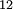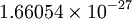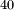Difference between revisions of "Atomic mass units"

The Atomic Mass Unit is defined as the twelfth part of the mass of a C$_{12}$ atom. Its value (in SI units, CODATA value) is$1.66054\times10^{-27}$ kilograms
Hence, it is approximately equal to the mass of a proton. Elements are asigned masses in these units which are not integer numbers. This is because neutrons and protons have slightly different masses, because nuclear binding forces cause the total mass of a nucleum to be less than that of the sum of its constituents, and, mainly, because of the appearance of natural isotopes. Thus, Potassium has a mass of 39.10 mainly because of natural K$^{40}$ isotopes.